## Mathematics Paper 1 Questions and Answers - Momaliche Post Mock 2020 Exam

INSTRUCTION TO CANDIDATE’S:

• This paper consists of TWO sections: Section I and Section II.
• Answer ALL the questions in Section I and any five questions from Section II.
• Answers and working must be written.
• Marks may be given for correct working even if the answer is wrong.
• Non-programmable silent electronic calculators and KNEC Mathematical tables may be used, except where stated otherwise.

SECTION I: (50 MARKS)
Answer all the question in this section in the spaces provided:

1. Evaluate                   (3mks)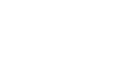2. Use square roots, reciprocal and square tables to evaluate to 4 significant figures the expression;
(0.06458)½ + (    2    )2      (4mks)
(0.4327)
3. Three similar 21-inch television sets and five similar 17-inch television cost Ksh.129,250. The difference between the cost of two 21-inch television sets and four 17-inch television sets is Ksh. 22,000. Calculate the price of a 21- inch television set and that of 17-inch television set. (3mks)
4. Simplify: (3 marks)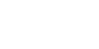5. Solve for x in the equation. (3 Marks)
9(x-1) x 3(2x+1) = 243
6. A classroom measures ( x + 2) m by ( x – 5)m. If the area of the classroom is 60m2.Find its length. (3 mks )
7. A tourist exchanged X US dollars for Kenya shilling when he arrived in Kenya. He spent three days in the country and paid Ksh 45,600 for expenses. He later left the country and exchanged the remainder back to US dollars. He went back with 1200 dollars. Find the value of X to the nearest dollar.                      (3mks)
Buying 1 US dollar = Ksh.98.36
Selling 1US dollar = Ksh.98.54
8. Three similar pieces of timber of length 240cm, 320cm and 380cm are cut into equal pieces. Find the largest possible area of a square which can be made from any of the three pieces. (3mks)
9. A regular polygon is such that its exterior angle is one eighth the size of interior angle.
Find the number of sides of the polygon. (3 mks)
10. Given that sin (2θ + 30) = Cos (θ − 60). Find the value of tan θ to two decimal places.
(2 mks)
11. The figure below shows a histogram.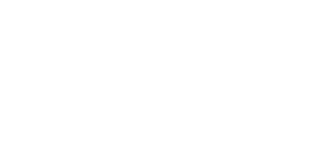Fill in the table below the missing frequencies. (3mks)
 Length in x cm Frequency 7.5≤ x ≤ 9.5 12 9.5≤ x ≤ 11.5 11.5≤ x ≤ 15.5 15.5≤ x ≤ 21.5
12. A straight line L1 is perpendicular to another line L2 whose equation is 3y+4x=12. If the two lines meet at point P which lies on the x-axis, find:
1. The co-ordinate of point P (1mk)
2. The equation of line L1 in the form y=mx+c (3mks)
13. The heights of two similar pails are 12cm and 8cm. The larger pail can hold 2 litres. What is the capacity of the smaller pail? Give your answer to 2 decimal places. (3mks)
14. Two matrices A and B are such that A= (k 4 3 2) and B = (1 3 3 −4) Given that the determinant of AB = 10, find the value of k. (3mks)
15. 1000cm3 of milk was shared by three children, Nick, Soita and Swalleh in the ratio
¼: ½ : 1/5,What volume did Soita get: (2mks)
16. Given that log a = 0.30 and log b = 0.48 find the value of log b(2 mks)                                                                                                                      a

SECTION II
Answer any five questions in this section.

1. The distance between two towns A and B is 760 km. A minibus left town A at 8:15a.m and traveled towards B at an average speed of 90km/h. A matatu left B at 10:35a.m and on the same day and travelled towards A at an average speed of 110km/h.
1.
1. How far from A did they meet? (4mks)
2. At what time did they meet? (2mks)
2. A motorist starts from his home at 10:30 a.m on the same day and traveled at an average speed of 100km/h. He arrived at B at the same time as the minibus. Calculate the distance from B to his home. (4mks)
2. The coordinates of a triangle ABC are A(1, 1) B(3, 1) and C (1, 3).
1. Plot the triangle ABC. (1 mark)
2. Triangle ABC undergoes a translation vector 2 2 . Obtain the image of A' B' C ' under the transformation, write the coordinates of A' B' C'. (2 marks)
3. A' B' C' undergoes a reflection along the line X = 0, obtain the coordinates and plot on the graph points A" B" C", under the transformation (2 marks)
4. The triangle A" B" C" , undergoes an enlargement scale factor -1, centre origin. Obtain the coordinates of the image A'" B"' C"'. (2 marks)
5. The triangle A"' B"' C"' undergoes a rotation centre (1, -2) angle 1200. Obtain the coordinates of the image Aiv Biv Civ. (2 marks)
6. Which triangles are directly congruent. (1 mark)
3. The income tax rates in a certain year are as shown below.
 Income (k₤ – p.a Rate (KSh. per ₤) 1 – 4200 2 4201 – 8000 3 8001 – 12600 5 12601 – 16800 6 16801 and above 7
Omar pays Sh. 4000 as P.A.Y.E per month. He has a monthly house allowance of KSh.10800 and is entitled to a personal relief of KSh. 1,100 per month. Determine:
1. his gross tax per annum in Kshs (2 Marks)
2. his taxable income in K₤ per annum (2 marks)
3. his basic salary in Ksh. per month (2marks)
4. his net salary per month (2 marks)
4. The diagram below shows two circles, centre A and B which intersect at points P and Q.
Angle PAQ = 70°, angle PBQ = 40° and PA = AQ = 8cm.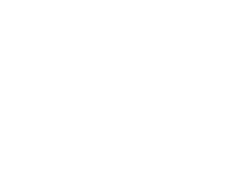Use the diagram to calculate
1. PQ to correct to 2 decimal places (2 Marks)
2. PB to correct to 2 decimal places (2 Marks)
3. Area of the minor segment of the circle whose centre is A (2 mks)
4. Area of the minor segment of the circle whose centre is B (2mks)
5. Area of the shaded region. (2mks)
5. Three Kenyan warships A, B and C are at sea such that ship B is 450km on a bearing of 0300 from ship A. Ship C is 700km from ship B on a bearing of 1200. An enemy ship D is sighted 1000km due south of ship B.
1. Taking a scale of 1cm to represent 100km locate the position of the ships A, B, C and D.      (4 Marks)
2. Find the compass bearing of:
1. Ship A from ship D (1 Mark)
2. Ship D from ship C (1 Mark)
3. Use the scale drawing to determine
1. The distance of D from A (1 Mark)
2. The distance of C from D (1 Mark)
4. Find the bearing of:
1. B from C (1 Mark)
2. A from C (1 Mark)
6. The figure below shows triangle OPQ in which OS = 1/3 OP and OR = 1/3 OQ. T is a point on QS such that QT = 3/4 QS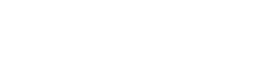1. Given that OP = p and OQ = q, express the following vectors in terms of p and q.
1. SR (1 Mark)
2. QS (2 Marks)
3. PT (2 Marks)
4. TR (2 Marks)
5. show that point PTR are collinear. (3mks)
7. In the figure below DA is a diameter of the circle ABCDE centre O. TCS is a tangent to the circle at C, AB = BC and angle DAC = 38°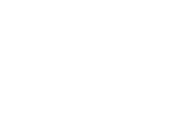Giving reasons, determine the following angles:
1. ∠DCT        (2 marks)
2. ∠DEA        (2 marks)
3. ∠ACB        (2 marks)
4. ∠BDC        (2 marks)
5. ∠BOA        (2 marks)
8.
1.
1. Fill the table below for the function.
y = 2x2 + 5x – 12 for − 8≤ x ≤ 4                   (2 marks)
 x −8 −7 −6 −5 −4 −3 −2 −1 0 1 2 3 4 2x2 128 18 2 32 5x −40 −15 5 20 −12 −12 −12 −12 −12 y 76 −9 −5 40
2. Using the table, draw the graph of the function y = 2x2 + 5x – 12. Use the scale 1cm to 1 unit on the x-axis and 1cm for 10 units for the y – axis
(4 marks)
2. Use the graph drawn above to solve the following equations.
1. 2x2 + 5x – 12 = 0         (2 marks)
2. 3 – 7x – 3x2 = 0            (2 marks)## MARKING SCHEME

1.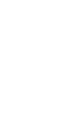2. √(6.458 ×10−2) + 2 (1/0.43272)2
2.541 + (2.311)2
= 2.541 + 2(5.307)
= 2.541 + 10.61
=13.155
3. x + 5y = 129250
2x - 4y = 22000
6x + 10y = 258500−
6x − 12y = 66000
22y = 192500
y = 8750
x = 28,500
4.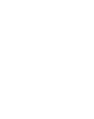5. 9(x-1) x 3(2x+1) = 243
Change to base 3
32(x-1)x 3(2x+1) = 35
2 (x – 1) + 2x + 1 = 5
2x – 2 = 2x + 1 = 5
4x – 1 = 5
4x = 6
x = 1.5
6. (x + 2 ) ( x – 5 ) = 60
x2 – 5x + 2x – 10 = 60
x2 – 3x – 70 = 0
x2 – 10x + 7x – 70 = 0
x ( x – 10) + 7(x – 10) = 0
( x – 10) ( x +7) = 0
x = 10 x = -7
Length 10 + 2 = 12m
7. 98.54 x 1200 = 118248
45600 + 118248
= 163848÷98.36 =1665.7991 = 1666 dollars
8. HCF of 240, 320 and 380
 120 320 380 2 120 160 190 2 60 80 95 5 12 16 19
HCF = 2 x 2 x 5 = 20 cm
Area = 202 = 400cm2
9. 1/8x + x = 180
9/8x = 180
x = 180 x 8
9
= 160
Exterior ˂ = 18 x 160 = 20
No. of sides 360 = 18
20
10. 2θ + 30 + θ - 60 = 90
3θ = 120
θ = 40
Tan 40 = 0.81
11. 12/2 = 1.2 x k
k =   = 5
1.2
 Length in x cm Frequency 7.5≤ x ≤ 9.5 12 9.5≤ x ≤ 11.5 5 x 1.6 = 16 11.5≤ x ≤ 15.5 5 x 0.8 x 4 = 16 15.5≤ x ≤ 21.5 5 x 2 x 6 = 60

12.
1. at P y= o ∴ 3(0) + 4(x) = 12
x = 3
= P(3,0)
2. y= −4/3 x + 3       m1 = −4/3 m2 =3/4
y – 0 = 3/4
x−3
4y = 3x – 9
∴  Y = 3/4x– 21/4
13. L. S. F= 12 : 8 = 3 : 2
V.S. F = 27 : 8
27 = 2litres
8 =      ?
8  x 2= 16 litres
27         27
(16/27 x 1000) cm3
= 592.592593cm=592.59cm3
14. AB= (K 4 3 2)  (1 2 3 −4)  = (K+12 2K−16 9 −2)
−2(K+12) −9 (2K−16)=10
−2K−24−18K+144=10
−20K = −110
∴ K=5.5
15. Total ration ¼ + ½ + 1/5 = 5+10+4 = 19
20         20
Vol. Shared = 19 x 1000 = 950cm3
20
Soita =  ½   x 950
19/20
10 x 950 = 500cm3
19
16. log b2 = log b2 − log a
a
2(0.48) – 0.30
0.96 – 0.30
= 0.66
17.
1. V.R = 90+110 = 200km/h
10.35a.m – 8.15a.m = 2hrs 20min = 2 1/3 hrs
Distance covered by matatu by 10.35cm
= 7/3 x 90 = 210km
Remaining distance 760 – 210 = 550km
Time taken = 550 = 2 ¾ hrs
200
Distance minibus had moved = 11/4 x 90 = 247.5km
Distance from A = 210 + 247.5
= 457.5km
2. They met at   10.35
+   2.45
13.20
= 1320hrs or 1:20pm
3. By 10.30a.m minibus had covered
9/4 x 90 = 202.5km
Time taken by minibus to B
760 – 202.5 = 67/36hrs = 6.19hrs
90
Distance covered by the motorist in this time.
6.19 x 100 = 619km

18.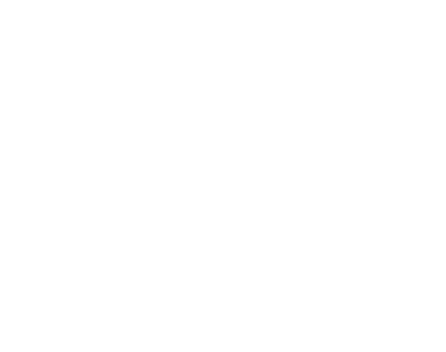AI = (1 1) + (2 2) = (3 3)
BI = (3 1) + (2 2) = (5 3)
CI = (1 3) +(2 2) = (3 5)
AII =(−5,3)  BII = (−3, 3) CII = (−3,5)
AIII = (3,−3) BIII = (5, −3) CIII = (3,−5)
AIV = (−0.6, −3.8) BIV =( −2, −2.8) CIV = (−3,−5)
Object and image 1, image 3, image 4,
1. (4000 x 12) + (1100 x 12)
= Sh. 61,200
2. 1st slab 4200 x 2= 8400
2nd slab 3800 x 3 = 11400
3rd slab 4600 x 5 = 23000 = 42800
4th slab y x 6 = (61,200 – 42800)
y = K₤ 3066.67
Taxable income= (12600 + 3066.67)
= K₤ 15666.67
3. 15666.67 x 20 − 10800
12
= KSh. 15,311.12
4. 26111.12 – 4000
= Sh. 22,111.12
19.
1. PQ = 2(8sin 35)
= 9.177 = 9.18
2. =8sin 35
sin 20
= 13.42
3. Area of minor segment circle centre A
70/360 x 3.142 x 8 x 8 −½ x 8 x 8sin 70 = 9.03
4. Area of minor segment circle centre B
40/360 x 3.142 x 13.422 –½ x 13.42 x 13.42 x sin 40
= 4.99cm2
5. Area of shaded region = Area of Δs
APQ and PQB – Area =(30.07+57.88)−(9.03+4.99)
=73.93cm2
20.
1.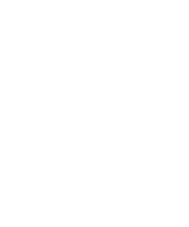2.
1. N20°W
2. S42°W
3.
1. 6.4 x 100km = 640km
2. 8.8 x 100km = 880km
4.
1. 300°
2. 268°

21.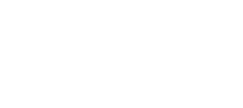OS = 1/3OP QT = ¾OS  OR = 1/3OQ
1. SR = SO + OR
=1/3P + 1/3Q
= 1/3(Q – P)
2. QR = QO + OS
= Q + 1/3P
= 1/3P – Q
3. PT = PS + ST
= −2/3P + ¼(−QS)
= −2/3p + ¼ (Q − 1/3P)
=−2/3 + ¼q − 1/12p
= −2/3 − 1/12p + ¼q
=−9/12p + ¼q
= ¼q − ¾p
4. TR = TQ + QR
= −¾ QR − 2/3Q
= −¾(1/3p – q)− 2/3Q
= −¼p + ¾q − 2/3q
9Q-8Q
12
= −¼P + 1/12Q
= 1/12Q −¼P
PT = ¼Q − ¾P
TR = 1/12Q −¼P
∴ PT = 3TR or 1/3PT = RT
Common point T
PT is a multiple of TR
PT = 3TR
Hence PTR are collinear
22.
1. ∠DCT = 38°-the angle which the chord makes with tangent is equal to the angle subtended by the same chord in the alternate segment of the circle
2. ∠DEA = 90°-Diameter subtends right angle at any point on the circumference of the circle
3. ∠ACB = 26°- The base angles of isosceles triangle are equal
4. ∠BDC = 26°-Angles subtended on the circumference by the same arc in the same segment are equal
5. ∠BOA = 52°-The angle which an arc subtends at the centre is twice that it subtends at any point on the circumference of the circle
23.

1.  x −8 −7 −6 −5 −4 −3 −2 −1 0 1 2 3 4 2x2 128 98 72 50 32 18 8 2 0 2 8 18 32 5x −40 −35 −30 −25 −20 −15 −10 −5 0 5 10 15 20 −12 −12 −12 −12 −12 −12 −12 −12 −12 −12 −12 −12 −12 −12 y 76 51 30 13 0 −9 −14 −15 −12 −5 6 21 40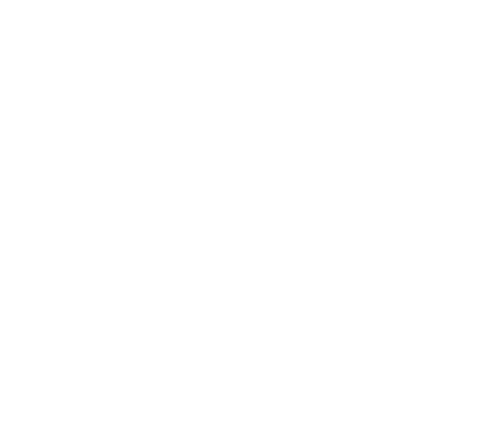B1 – For half + correct values B1B1 for all values.
2.
1. 2x2 + 5x – 12 = y
2x2 + 5x – 12 = 0
0 = y
x = −4 or 1.5
2. (2x2 + 5x – 12 = y)3
(3x2 + 7x –3 = 0)2
(6x2 +15x – 36 = 3y) − (6x2 + 14x – 6 =0)
x – 30=3y
y=1/3x-10
x=–4.6, 2.4

• ✔ To read offline at any time.
• ✔ To Print at your convenience
• ✔ Share Easily with Friends / Students